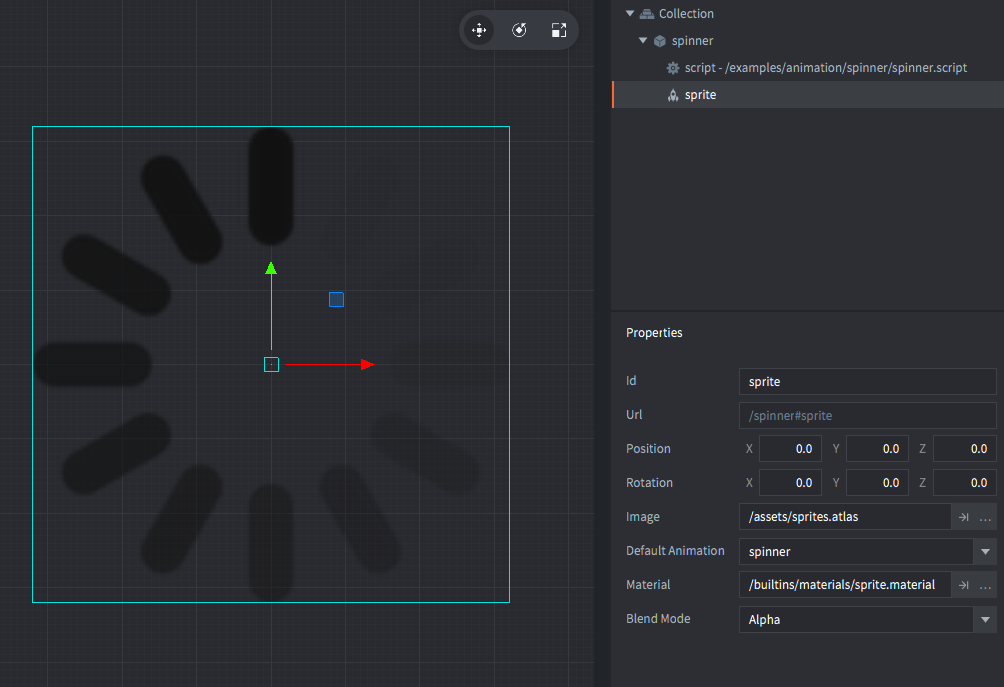#### Setup#### Scripts

spinner.script

```function init(self)
self.t = 0 -- <1>
self.speed = 16 -- <2>
end

function update(self, dt)
self.t = self.t + dt -- <3>
local step = math.floor(self.t * self.speed) -- <4>
local angle = math.pi / 6 * step -- <5>
local rot = vmath.quat_rotation_z(-angle) -- <6>
go.set_rotation(rot) -- <7>
end

--[[
1. Store a timer value (seconds elapsed) in the current script component (accessed through `self`).
2. A speed value. How many rotation steps to perform each second.
3. Increase timer value with the delta time elapsed since last `update()`.
4. Calculate which step to rotate to.
5. Calculate rotation angle (in radians) based on which step to rotate to.
6. Create a rotation quaternion with `angle` rotation around the Z axis.
7. Set the rotation on the current game object.
--]]```

If you want to play with these examples, you can get the project on Github.

Do you want to see more examples? Why not write a few yourself and submit a pull request? We love contributions.